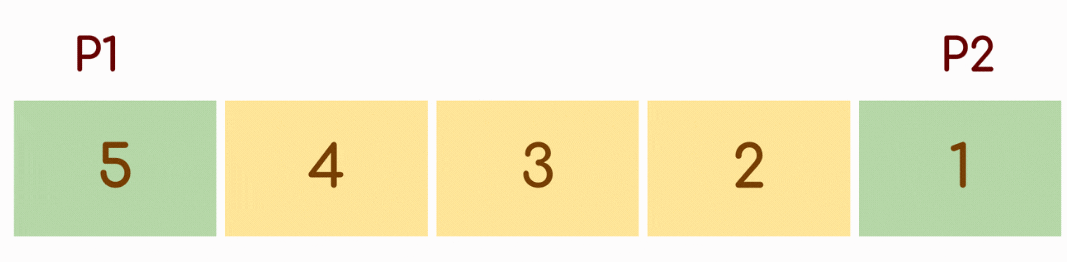# Reverse array in C

Problem Statement: Given an array, reverse the given array. Reverse array in C.

Examples:

```Example 1:
Input: arr[] = 1 2 3 4 5, n = 5
Output: 5 4 3 2 1

Example 2:
Input: arr[] = 5 4 3 2 1, n =5
Output: 1 2 3 4 5```

DisclaimerDon’t jump directly to the solution, try it out yourself first.

### Approach 1:

Before we think about the approach, can you print the array in reverse order if you know the size of the array?

It’s easy right? Simply run a for loop from last element to first element and print the elements simultaneously.

Now, how can you use this to reverse the array?

Steps:

• Create an auxiliary array or additional temporary array of same size as input array.
• Traverse the input array from end and auxiliary array from start.
• Simultaneously keep copying elements from end of input array to start of auxiliary array.
• At the end of loop auxiliary array will be the reversed array.

Finally, copy all elements of auxiliary array to input array.

## Approach 2:

Can you improve the approach 1? What if you are not allowed to create an auxiliary array?

If you observe carefully, to reverse an array, you just to need keep on swapping the elements from both directions(front and back) until you reach the middle element.

For example:

```Input Array, arr[] = {1,2,3,4,5,6,7}

Swap first and last element:
arr[] = {7,2,3,4,5,6,1}

Swap second and second-last element:
arr[] = {7,6,3,4,5,2,1}

Swap third and third-last element:
arr[] = {7,6,5,4,3,2,1}

Now, since we have reached mid, no need to swap.
The array arr[] is now reversed.```
• Declare two variables start and end.
• Initialize start = 0 and end = n-1 where n is the length of the array.
• swap arr[start] and arr[end]
• keep increasing start = start + 1 and decreasing end = end -1 until start > end
• and print the reversed arrayCode:

## C Program

``````// Iterative C program to reverse an array
#include<stdio.h>

void reversee(int arr[], int start, int end)
{
int temp;
while (start < end)
{
temp = arr[start];
arr[start] = arr[end];
arr[end] = temp;
start++;
end--;
}

}

int main()
{
int arr[] = {1, 2, 3, 4, 5, 6};
int n = sizeof(arr) / sizeof(arr);

reversee(arr, 0, n-1);
printf("Reversed array is \n");
for(int i=0;i<n;i++)
{
printf("%d ",arr[i]);
}
return 0;
}
``````

Output:

Reversed array is

6 5 4 3 2 1

Time complexity: O(n), where n is the length of the array

Space Complexity: O(1)

Special thanks to Subhrajit Das for contributing to this article on takeUforward. If you also wish to share your knowledge with the takeUforward fam, please check out this article